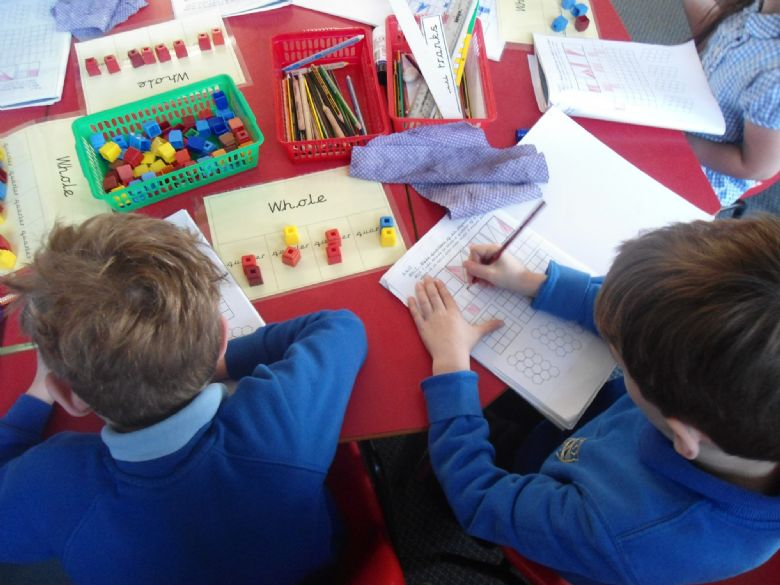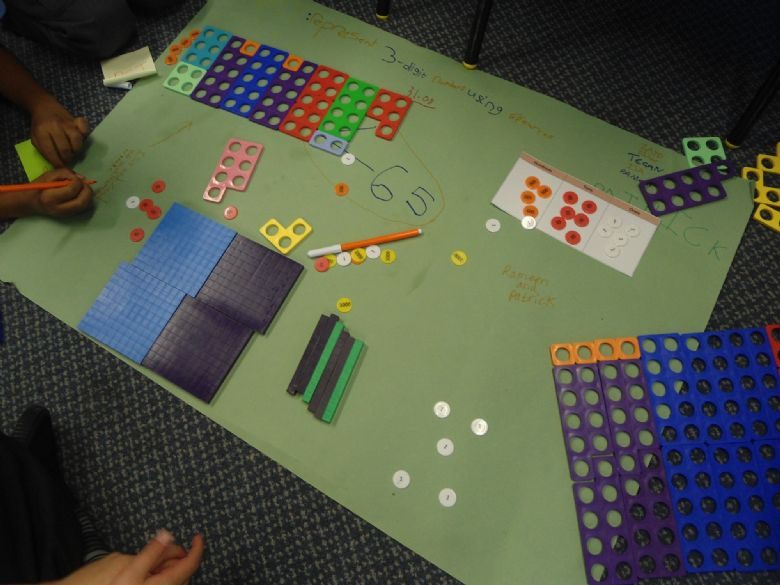# Little Hill Primary School# Maths

Intent

We believe that maths is essential to everyday life and that a high quality mathematics education provides a foundation for understanding the world, the ability to reason and a sense of enjoyment and curiosity for the subject.  Our aim are for all pupils:

• To become fluent in the fundamentals of mathematics, through varied and frequent practise so that they are able to recall and apply what they have learnt quickly and accurately.
• Are able to reason mathematically by following a line of enquiry, conjecturing relationships and generalisations and developing an argument, justification or proof, using mathematical language.
• Can solve problems by applying their mathematics to a variety of problems.

We deliver a full curriculum from EYFS up to Year 6, which caters for the needs of all individuals. This provides pupils with the necessary skills and knowledge to be successful at secondary school and beyond

Implementation

• Pupils are taught mathematics through the domains of number, measurement, geometry and statistics through the 2014 National Curriculum.
• EYFS pupils are taught through the 2021 Statutory Framework for the early years foundation stage.  Maths is a specific area and our youngest children develop the building blocks to excel mathematically.
• Teachers plan using the White Rose Scheme of Work to sequence the learning into blocks of progressive, cohesive steps to ensure that the whole curriculum in covered.
• The school follows a mastery approach which focuses on 5 big ideas: coherence, representation and structure, (concrete, pictoral, abstract approach), mathematical thinking, fluency, variation.
• Basic Maths skills are taught daily. Focussing on key mathematical skills including place value, the four operations and fractions
• A range of reasoning resources are used to challenge all children and give them the opportunity to reason with their understanding.
• Children are given the opportunity to solve problems in a range of contexts, to understand the importance of maths in the wider world.
• Lessons begin with a review of previous learning before beginning new learning.
• Children are given frequent opportunities to talk throughout the lesson with each other, sharing their own ideas and stem sentences are modelled by the teacher.
• Pupils understand that making mistakes is okay. They have the confidence to ‘have a go’, develop resilience and have a growth mindset to persevere.
• All pupils are expected to achieve the same learning however the depth will be varied. Some pupils will have scaffolding. Teachers have high expectations and all children are expected to succeed and make progress from their different starting points.
• Pupils are given adequate opportunities to practise and consolidate their learning before moving on to the next step.
• Gaps are identified quickly and intervention is rapid through ‘grab and go’ sessions.
• Learning environments are used to support pupils in lessons, including interactive working wall displays.
• Homework is set regularly to review children's learning.
• Where possible, links are made between mathematical domains, with other subjects across the curriculum and to the wider world.
• Teachers continually monitor and assess individual pupils’ understanding and progress against the expected standards for their year group.

Impact

At Langmoor you will see:

• Pupils achieve to the best of their ability and reaching their potential in maths.
• Pupils understand the relevance and importance of what they are learning and know that maths is essential to everyday life.
• Pupils have a positive attitude towards maths and their own learning.
• Pupils have a good awareness of their strengths and targets for development.
• Confident children who can all talk about Maths and their learning and the links between Mathematical topics.
• Pupils show mastery in all the domains of maths, having a secure long term, deep and adaptable understanding of concepts that they can apply to different contexts.
• Pupils demonstrate quick recall of facts and procedures.
• Teachers with good subject knowledge who know their children well and what they need to be learning next.
• Lessons make the best use of resources to support learning.
• Books evidence work of a high standard which children take pride in.Please see our new calculations policy detailed below for each year group.Video for parents and children - Miss Payne is modelling how to use the part/part whole method in Maths:

https://whiterosemaths.com

The table below shows the progression of multiplication and division across the school.

 Year 2 Recall and use division facts for the 2, 5 and 10 multiplication tables.  Show that multiplication can be done in any order, but division cannot.  Solve problems involving multiplication and division using arrays, repeated addition and known facts. Year 3 Recall and use division facts for the 3, 4 and 8 multiplication tables.  Multiply a two digit number by a one digit number using mental and informal written methods.  Solve problems involving missing number problems, involving multiplication and division including scaling and correspondence problems. Year 4 Recall and use division facts for the multiplication tables up to 12 x 12.  Use place value, known and derived facts to multiply and divide mentally.  Multiply two digit and three digit numbers by a one digit number using a more formal written layout. (Column method).  Solve problems involving multiplication and division including scaling and correspondence problems. Year 5 Identify multiples, square numbers, cube numbers, factors, prime numbers and composite (non prime) numbers.  Multiply and divide numbers mentally using known facts.  Multiply up to 4 digits by a one or two digit number using formal written methods such as long multiplication.  Divide up to 4 digits by a one digit number using formal written methods such as short division and interpret remainders appropriately for the context.  Multiply and divide whole numbers and decimals by 10, 100 and 1000.  Solve problems involving multiplication and division, using their knowledge of factors, multiples, squares and cubes.  Solve problems involving scaling by simple fractions and problems involving simple rates. Year 6 Identify common factors, common multiples and prime numbers.  Use estimation to check answers to calculations and determine an appropriate degree of accuracy.  Multiply up to 4 digits by a two digit using long multiplication.  Divide up to 4 digits by a two digit number using long division and short division and interpret remainders as whole number remainders, fractions or by rounding, if appropriate.

Your child will complete a 'Multiplication Check' at the end of year 4 'to determine whether pupils can recall their times tables fluently, which is essential for future success in mathematics'.

At Langmoor, we recognise the importance of quick recall of multiplication and division facts. we have subscribed to TT Rock Stars which is a carefully sequenced programme to consolidate and practise times tables.